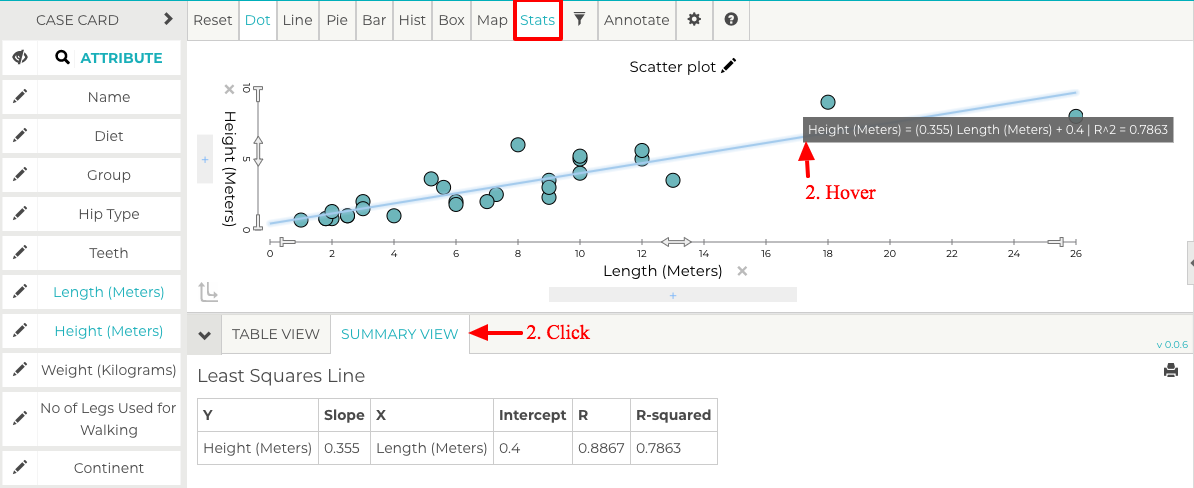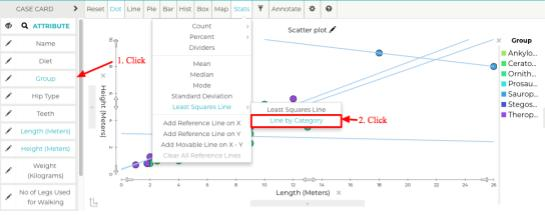# Least Squares Line

Tuva's data and graphing tools offer teachers and students the ability to analyze their data using the least squares line, also known as the line of best fit.

Add a Single Least Squares Line

Complete the following steps to add a least squares line to a scatterplot and view the associated equation.

Steps

1. Once you have dragged and dropped a numerical attribute on each axis, the next thing you'll need to do from the Stats menu is select the Least Squares Line option.
2. Now that the least squares line is added to the plot, you may view the equation and its components in one of two ways. Either by hovering on the line to view the equation or by opening the Summary View and seeing its components listed in the table.Adding a Least Squares Line by Category

Within a scatterplot, Tuva allows you to separate data points by category. Additionally, you can generate a least squares line for each of these categories. Complete the following steps to add a least squares line for each category in a scatterplot and view the associated equations.

Steps

1. Once you have dragged and dropped a numerical attribute on each axis, select a categorical attribute by single clicking it in the case card.
2. Hover your mouse over "Stats" to reveal the dropdown menu and click "Least Squares Line by Category."
3. Now that the least squares line is added to the plot, you may view the equation and its components in one of two ways. Either by hovering on the line to view the equation or by opening the Summary View and seeing its components listed in the table.